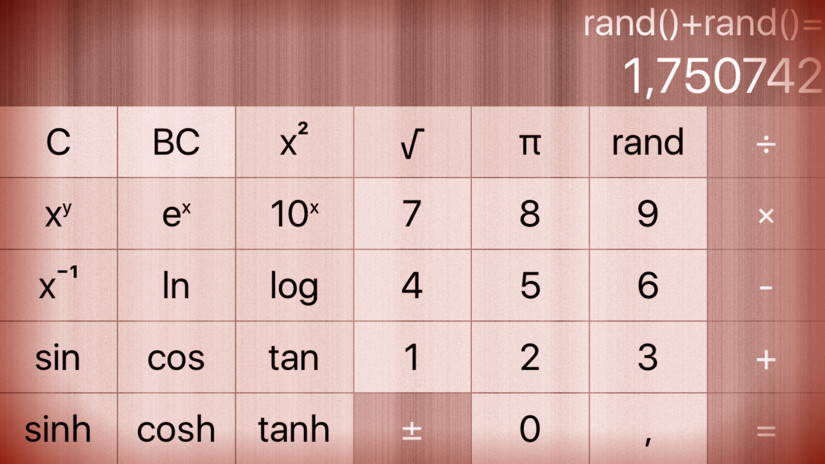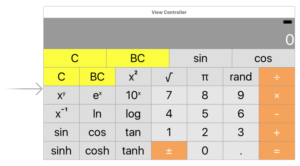# cs193p – Assignment #1 Extra Task #3Make one of your operation buttons be “generate a random double-precision floating point number between 0 and 1”. This operation button is not a constant (since it changes each time you invoke it). Nor is it a unary operation (since it does not operate on anything). Probably the easiest way to generate a random number in iOS is the global Swift function arc4random() which generates a random number between 0 and the largest possible 32-bit integer (UInt32.max). You’ll have to get to double precision floating point number from there, of course.

Change one of the buttons in story board:Add an operation without operands to the the calculator model:

```    private enum Operation {
...
case nullaryOperation(() -> Double, String)
...
}
```

The “random” operation returns the function generating the random number as well as a string for its description:

```private let operations: Dictionary<String,Operation> = [
...
"rand" : Operation.nullaryOperation(
{ Double(arc4random()) / Double(UInt32.max) },
"rand()"
)
]
```

… which are used to calculate the result and the description:

```    mutating func performOperation(_ symbol: String) {
...
case .nullaryOperation(let function, let description):
accumulator = (function(), description)
...
}
```

The complete code for the assignment #1 extra task #3 is available on GitHub.